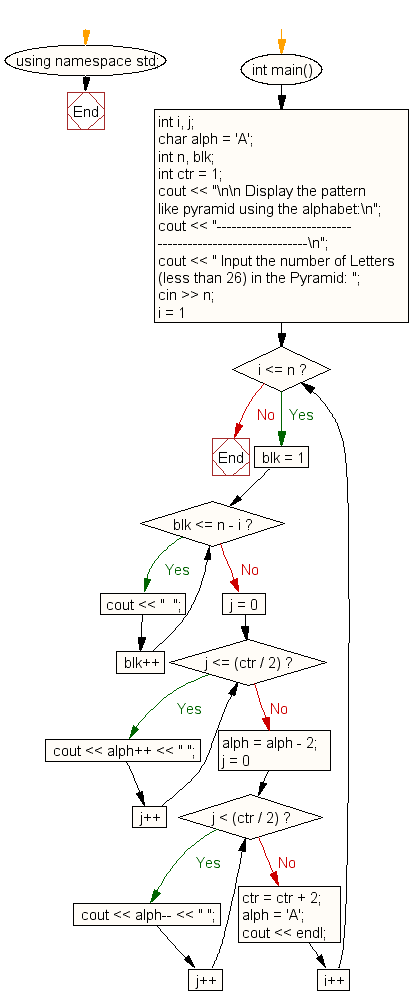﻿ C++ : Show a pattern like pyramid using the alphabet

# C++ Exercises: Display the pattern like pyramid using the alphabet

## C++ For Loop: Exercise-48 with Solution

Write a program in C++ to display the pyramid pattern using the alphabet.

Sample Solution:

C++ Code :

``````#include <iostream>
using namespace std;

int main()
{
int i, j;
char alph = 'A';
int n, blk;
int ctr = 1;
cout << "\n\n Display the pattern like pyramid using the alphabet:\n";
cout << "---------------------------------------------------------\n";
cout << " Input the number of Letters (less than 26) in the Pyramid: ";
cin >> n;
for (i = 1; i <= n; i++) {
for (blk = 1; blk <= n - i; blk++)
cout << "  ";
for (j = 0; j <= (ctr / 2); j++)
{
cout << alph++ << " ";
}
alph = alph - 2;
for (j = 0; j < (ctr / 2); j++)
{
cout << alph-- << " ";
}
ctr = ctr + 2;
alph = 'A';
cout << endl;
}
}
``````

Sample Output:

``` Display the pattern like pyramid using the alphabet:
---------------------------------------------------------
Input the number of Letters (less than 26) in the Pyramid: 5
A
A B A
A B C B A
A B C D C B A
A B C D E D C B A
```

Flowchart:C++ Code Editor: